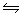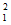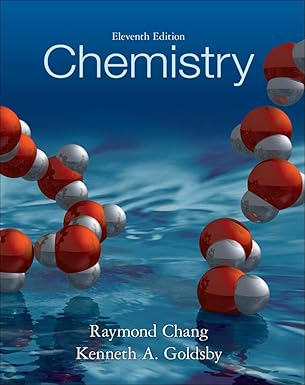Top Posters
Since Sunday
o
10
j
9
m
9
C
8
s
8
a
8
f
8
y
8
n
8
d
8
M
7

# Consider the two gaseous equilibria:SO2(g) + 1/2O2(g) SO3(g)K12SO3(g) SO2(g) + O2(g)K2The values of ...

wrote...
Posts: 102
Rep:
A month ago
Consider the two gaseous equilibria:
 SO2(g) + 1/2O2(g)SO3(g) K1 2SO3(g)SO2(g) + O2(g) K2
The values of the equilibrium constants K1 and K2 are related by

▸ K2 =  K12

▸ K22 = K1

▸ K2 = 1/K▸ K2 = 1/K1

▸ none of these.
Textbook## ChemistryEdition: 11thAuthors:
Replies
Answer verified by a subject expert
voltayrevoltayre
wrote...
Posts: 121
Rep:
A month ago

### Related Topics

westleafs Author
wrote...

A month ago
 Helped a lot
wrote...

Yesterday
 This helped my grade so muchwrote...

2 hours ago
 Thank you, thank you, thank you!
Explore